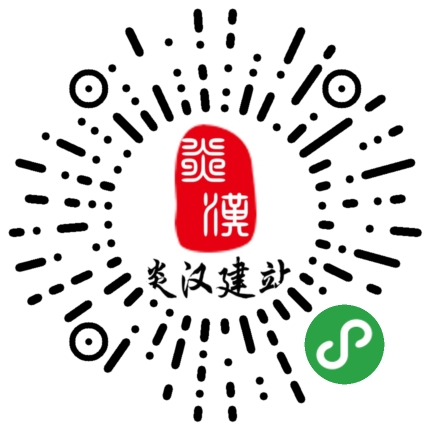#### 炎汉常用网址

 功能 免费版 企业版 高级版 空间大小 2G 5G 50G 赠送域名 × × × 独立域名绑定 × 3个 3个 二级域名绑定 1个 1个 1个 百度收录 × √ √ 手机微站 √ √ √ 网站备案支持 × √ √ 去除底标 × × × 子站登录 × √ √ 多语言支持 √ √ √ 境内服务器 √ √ √ 香港服务器 × √ √

#### 版本

 功能 免费版 企业版 高级版 会员概要 √ √ √ 会员等级 √ √ √ 会员卡 √ √ √ 会员积分 √ √ √ 充值消费 × × × 会员提现 × × × 会员设置 √ √ √ 会员地址 √ √ √ 浏览权限 √ √ √ 会员发布 × × × 会员中心 √ √ √ 会员信息及注册 √ √ √ 第三方登录 √ √ √
 功能 免费版 企业版 高级版 订单概要 × × √ 订单管理 × × √ 门店商铺统计 × × √ 退换货 × × √ 销售报表 × × √ 商城设置 × × √ 在线支付 × × √ 发票设置 × × √ 物流配送 × × √ 小票打印机 × × × 支付卡 × × × 三级分销 × × × 会员商铺 × × × 优惠券 × × √ 会员商城 × × × 满减 × × × 积分商城 × × √ 清仓购 × × × 团购 × × √ 限时购 × × × 一元购 × × ×
 功能 免费版 企业版 高级版 团队展示 × × √ 有问必答 × × √ 视频课程 × × × 网站评论 √ √ √ 论坛 × × √ 行业知道 √ √ √ 行业百科 √ √ √ 博客 √ √ √ 会议活动 × × √ 自定义表单 √ √ √ 门店设置 √ √ √ 万能查询 × × × 720VR全景 × √ √ 留言板 √ √ √ 站内广告 × √ √ 网站分享 √ √ √ 相册 √ √ √ 开篇动画 × √ √ 下载功能 × × √ 企业地标 √ √ √ 城市分站 × √ √
 功能 免费版 企业版 高级版 搜索优化 × √ √ 关键词向导 × × √ 网站地图 × √ √
 功能 免费版 企业版 高级版 在线客服 √ √ √ 背景音乐 √ √ √ 网站安全 √ √ √ 网站小图标 × √ √ 网站验证 × √ √ 短信提醒 × × × 访问统计 √ √ √

#### 温馨提示：行业功能组件只限O2O版本以上可开通，低于O2O全能版的网站暂不开放行业功能组件#### 关注炎汉建站微信#### Copyright @ 2014-2020.  商丘炎汉文化传播有限公司   炎汉建站   版权所有.   豫ICP备17032509号      客服QQ：40050141      客户服务热线：0370-7998889